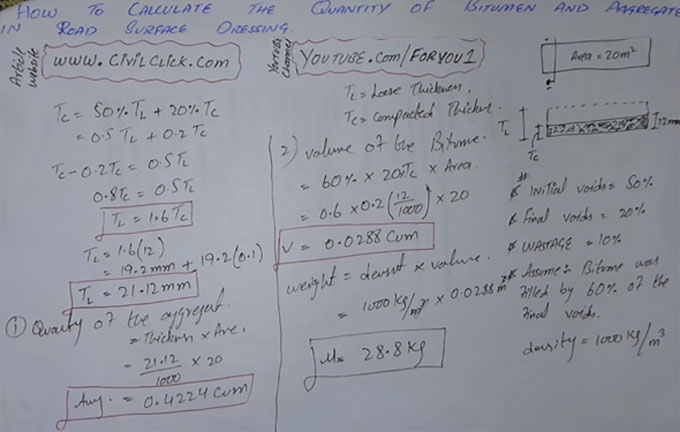# Step for determining the quantity of bitumen & aggregates for road surface dressingThis construction video tutorial sheds light on the detail method to workout the quantity of bitumen and aggregates toward road surface dressing (Top Layer of the road).

The area of the road is taken as, Area = 20m2
The density of the road surface dressing on top should taken as 12 mm and it is demoted as TC

Here, the value of TL is unknown. TL denotes loose thickness that should be maintained after compaction

TL is based on the following factors :-
Initial Voids = 50% (existence or air or air pockets i.e. gaps in aggregates)
Final Voids = 20%
Wastage = 10%
Suppose that Bitumen will fill 60% of the final voids.
TC is defined as compacted thickness

To determine the thickness of TL, the following formula is used :-
TC = 50% in TL + 20% in TC
Now convert the value to decimal by dividing with 100 = 0.5 TL + 0.2 TC

TC – 0.2 = 0.5 TL = 0.8 TC = 0.5 TL
TL = 1.6 TC
putting the value of compacted thickness, we get
TL = 1.6(12) = 19.2mm
So, loose depth is 19.2 mm

Now, after adding 10% wastage, we get = 19.2mm + 19.2(0.1) [convert to decimal value by dividing with 100]
= 21.12mm
So, loose thickness should be 21.12mm to attain 12mm compacted thickness

To get more details, watch the following video tutorial.

Video Source: F&U-FORYOU